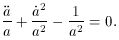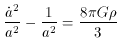3. The Open Model

The so-called Open Model (13) is the case where k = -1 and the geometry is said to be hyperbolic. Taking k = -1 in the Friedmann equations (29), (28),(39)(40)

Solving these equations (39), (40) yields the same value of the density (37) as the closed model, but in this case q < 1/2 and< 1, as previously discussed.

13 Although it is a standard practice to refer to this case (k = -1) as the `Open' Model, it should be noted that the model can actually correspond to a closed universe. This is the result of a non-trivial topology, which results in geometry that can be hyperbolic; but, the topology can cause it to be contained in a finite space [16, 17].